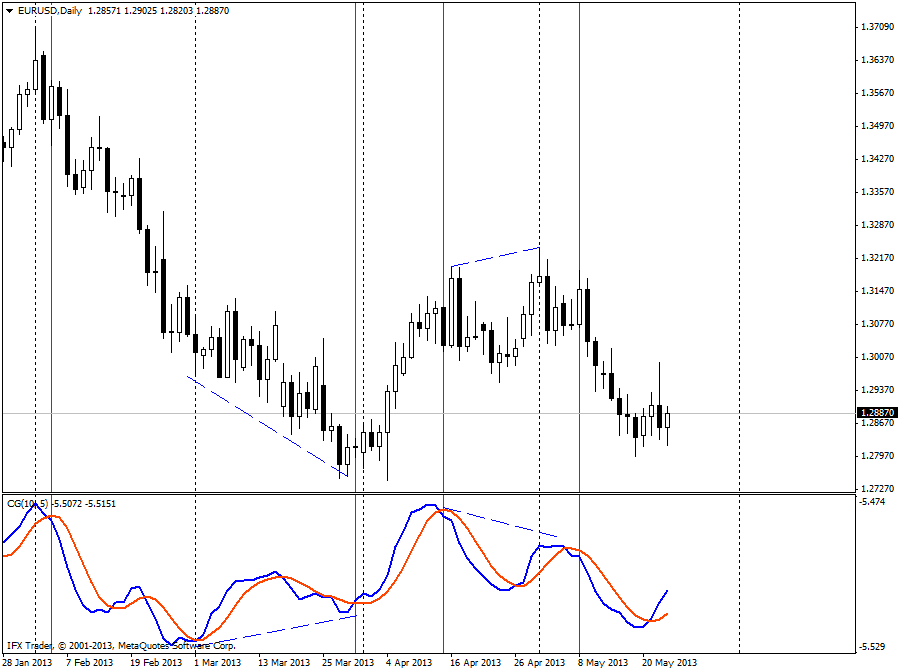Center of Gravity indicator

# Center of Gravity indicator

The Center of Gravity is an oscillator developed by John Ehler and presented in Stocks & Commodities magazine (05.2002). This oscillator produces almost zero lag indicating the pivot points with the precise accuracy. The indicator was the result of studies of adaptive filters.

#### Formula

CG = -1*NUM/DEN

NUM = int (n, i = 0) [PRICE{i}*{i+1}]

DEN = int (n, i = 0) [PRICE {i}], where

PRICE [i] = the price i bar (bars) back;

PRICE  = the current bar price;

PRICE  = the price 2 bars back.

The Center of Gravity indicator helps to identify the main pivot points with no lag which is the principle advantage of this tool for both technical analysis and trading.

After the lagging of various price filters with the final impulse response in accordance with the relative amplitude of filters coefficients was investigated, the idea of the Center of Gravity for MetaTrader appeared. The SMA can be such a filter where all the coefficients have the same value. As the result, the center of gravity for SMA is the exact center of a filter.

The Center of Gravity indicator resembles stochastic oscillator with the only difference that it has no overbought and oversold areas and its major signals form two signal lines (red and blue).

The crossing of signal lines serves as the indicator's major signal:

• If the red line crosses the blue one bottom up, the indicator generates a buy signal.
• In case the red line crosses the blue one to the downside, the indicator gives a sell signal.

Since the indicator was developed as less lagging indicator, such signals can be successfully applied in trading without any additional filter.

One more signal that can be generated by the Center of Gravity computer indicator is the classical signal of divergence:

• If the price has formed two new highs, (at that the second one is higher than the first one) and the Center of Gravity indicator did not show a new maximum, a divergence signal appears to warn of asset selling.
• If the price has formed two new lows (at that the first one is lower than the second one), while the Center of Gravity indicator did not produce a new maximum, a new convergence signal appears, contemplating an asset purchasing.Can't speak right now?